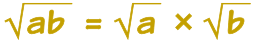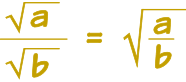# Simplifying Square Roots

To simplify a square root: make the number inside the square root as small as possible (but still a whole number):

### Example: √12 is simpler as 2√3

Get your calculator and check if you want: they are both the same value!

Here is the rule: when a and b are not negativeAnd here is how to use it:

### Example: simplify √12

12 is 4 times 3:

√12 = √(4 × 3)

Use the rule:

√(4 × 3) = √4 × √3

And the square root of 4 is 2:

√4 × √3 = 2√3

So √12 is simpler as 2√3

Another example:

### Example: simplify √8

√8 = √(4×2) = √4 × √2 = 2√2

(Because the square root of 4 is 2)

And another:

### Example: simplify √18

√18 = √(9 × 2) = √9 × √2 = 3√2

It often helps to factor the numbers (into prime numbers is best):

### Example: simplify √6 × √15

First we can combine the two numbers:

√6 × √15 = √(6 × 15)

Then we factor them:

√(6 × 15) = √(2 × 3 × 3 × 5)

Then we see two 3s, and decide to "pull them out":

√(2 × 3 × 3 × 5) = √(3 × 3) × √(2 × 5) = 3√10

## Fractions

There is a similar rule for fractions:### Example: simplify √30 / √10

First we can combine the two numbers:

√30 / √10 = √(30 / 10)

Then simplify:

√(30 / 10) = √3

## Some Harder Examples

### Example: simplify √20 × √5√2

See if you can follow the steps:

√20 × √5√2
√(2 × 2 × 5) × √5√2
√2 × √2 × √5 × √5√2
√2 × √5 × √5
√2 × 5
5√2

### Example: simplify 2√12 + 9√3

First simplify 2√12:

2√12 = 2 × 2√3 = 4√3

Now both terms have √3, we can add them:

4√3 + 9√3 = (4+9)√3 = 13√3

## Surds

Note: a root we can't simplify further is called a Surd. So √3 is a surd. But √4 = 2 is not a surd.